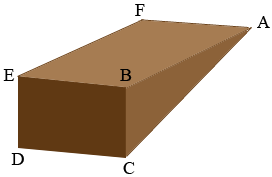SEARCH HOMEMath Central Quandaries & QueriesQuestion from Jasmine: what is the volume and surface area of a wedge with definitionHi Jasmine,

Is this what I see as a wedge? Let me know if know if it is something else.The surface can be see as 5 pieces, two congruent triangles (one I have labeled $ABC$), two long rectangles ($AFEB$ an one on the bottom, and one more rectangle $BEDC.$ The surface area of the wedge is the sum of the areas of the five pieces. If you know the dimensions then you can calculate the area of each rectangle. If you know the heights of the triangles you can find their areas using the fact that the area of a triangle is one half the base, times the height. If you don't know the heights of the triangles you might use Heron's Formula.

I am assuming that the angle $ABF$ is a right angle. In this case the volume of the wedge is the area of triangle $ABC$ time the length of $BE.$

PennyMath Central is supported by the University of Regina and The Pacific Institute for the Mathematical Sciences.